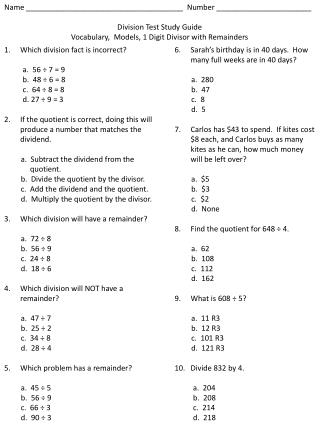DownloadDownload PresentationName ______________________________________ Number _______________________

# Name ______________________________________ Number _______________________

Télécharger la présentation## Name ______________________________________ Number _______________________

- - - - - - - - - - - - - - - - - - - - - - - - - - - E N D - - - - - - - - - - - - - - - - - - - - - - - - - - -
##### Presentation Transcript

1. Name ______________________________________ Number _______________________ Division Test Study Guide Vocabulary, Models, 1 Digit Divisor with Remainders Which division fact is incorrect? a. 56 ÷ 7 = 9 b. 48 ÷ 6 = 8 c. 64 ÷ 8 = 8 d. 27 ÷ 9 = 3 If the quotient is correct, doing this will produce a number that matches the dividend. a. Subtract the dividend from the quotient. b. Divide the quotient by the divisor. c. Add the dividend and the quotient. d. Multiply the quotient by the divisor. Which division will have a remainder? a. 72 ÷ 8 b. 56 ÷ 9 c. 24 ÷ 8 d. 18 ÷ 6 Which division will NOT have a remainder? a. 47 ÷ 7 b. 25 ÷ 2 c. 34 ÷ 8 d. 28 ÷ 4 Which problem has a remainder? a. 45 ÷ 5 b. 56 ÷ 9 c. 66 ÷ 3 d. 90 ÷ 3 Sarah’s birthday is in 40 days. How many full weeks are in 40 days? a. 280 b. 47 c. 8 d. 5 Carlos has \$43 to spend. If kites cost \$8 each, and Carlos buys as many kites as he can, how much money will be left over? a. \$5 b. \$3 c. \$2 d. None Find the quotient for 648 ÷ 4. a. 62 b. 108 c. 112 d. 162 What is 608 ÷ 5? a. 11 R3 b. 12 R3 c. 101 R3 d. 121 R3 Divide 832 by 4. a. 204 b. 208 c. 214 d. 218

2. 11. Which is the quotient of 81 divided by 7? a. 9 b. 10 R11 c. 11 R4 d. 12 Dan is putting his sports card collection in a scrapbook. He can fit 6 cards on each page. How many pages does Dan need for 28 cards? a. 4 b. 4 R4 c. 5 d. 5 R1 What does the letter n represent in this problem? 45 ÷ n = 9 a. Dividend b. Divisor c. Quotient d. remainder How do you check the answer when there is a remainder in the quotient? a. Multiply the quotient by the divisor. Then add the remainder to the product. b. Multiply the quotient by the divisor. Then subtract the remainder from the product. c. Add the remainder to the quotient. Then multiply the sum by the divisor. d. Subtract the remainder from the quotient. Then multiply the difference by the divisor.

3. Answers • A • D • B • D • B • D • B • D • D • B • B • C • C • B • A